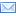#Difference Between Similar Terms and Objects

# Difference Between Equations and FunctionsEquations vs Functions

When students encounter algebra in high school, the differences between an equation and a function becomes a blur. This is because both use expressions in solving the value for the variable. Then again, the differences between these two are drawn by their outputs. Equations can have one or two values for the variables used depending on the value equated with the expression. On the other hand, functions can have solutions based on the input for the values of the variables.

When one solves for the value of “X” in the equation 3x-1=11, the value of “X” can be derived through the transposition of the coefficients. This then gives 12 as the solution of the equation. On the other hand, the function f(x)=3x-1 can have varied solutions depending on the assigned value for x. In f(2), the function can have a value of 5, while making it f(4) can give out the function’s value of 11.
In simpler terms, the value of an equation is determined by the value the expressions are equated with, while the value of a function depends on the value of “X” assigned.

To make it clearer, students should understand that a function gives the value and defines the relationships between two or more variables. For every value of “X” assigned, students can get a value that can describe the mapping of “X” and the function input. On the other hand, equations show the relationship between their two sides. The right side equated to a value or expression to the left side of the equation simply means that the value of both sides is equal. There is a definite value that would satisfy the equation.

Graphs of equations and functions also differ. For equations, the X-coordinate or the abscissa can take on different Y-coordinates or distinct ordinates. The value of “Y” in an equation can vary when the values of “X” changes, but there are cases when a single value of “X” can result in multiple and different values of “Y.” On the other hand, the abscissa of a function can only have one ordinate as the values are assigned.

Different tests are also applied in the precision assessments of equation and function graphs. The graph of an equation drawn using a single line for linear and parabola for higher-degree equations should only intersect at one point with a vertical line drawn in the graph.
The graph of a function, however, will cross the vertical line at two or more points.
Equations can always be graphed because of the definite values of “X” solved through transposition, elimination, and substitutions. As long as the students have the values for all the variables, it would be easy for them to draw the equation in a Cartesian plane. On the other hand, functions can have no graph at all. Derivative operators, for example, can have values that are not real numbers and, therefore, cannot be graphed.

These things being said, it is logical to infer that all functions are equations, but not all equations are functions. Functions, then, become a subset of equations that involve expressions. They are described by equations. Thus, putting two or more functions with a mathematical operation can form an equation such as in f(a)+f(b)=f(c).

Summary:

1.Both equations and functions use expressions.
2.Values of variables in the equations are solved based on the value equated, while values of variables in functions are assigned.
3.In a vertical line test, graphs of equations intersect the vertical line at one or two points, while graphs of functions can intersect the vertical line at multiple points.
4.Equations always have a graph while some functions cannot be graphed.
5.Functions are subsets of equations.

Latest posts by Celine (see all)

### Search DifferenceBetween.net :

Custom Search

Help us improve. Rate this post!(4 votes, average: 2.00 out of 5)Loading...Email This Post : If you like this article or our site. Please spread the word. Share it with your friends/family.

Wrong:

Different tests are also applied in the precision assessments of equation and function graphs. The graph of an equation drawn using a single line for linear and parabola for higher-degree equations should only intersect at one point with a vertical line drawn in the graph.
The graph of a function, however, will cross the vertical line at two or more points.

Correct:

An equation defines a function if each vertical line in a rectangular coordinate system passes through at most one point on the graph of the equation.
If any vertical line passes through two or more points on the graph of an equation, then the equation does not define a function.

2. error in second paragraph. solution to equation is 4, not 12. 3x = 12 so x = 4.

3. Thank you so much.

Please note: comment moderation is enabled and may delay your comment. There is no need to resubmit your comment.

Articles on DifferenceBetween.net are general information, and are not intended to substitute for professional advice. The information is "AS IS", "WITH ALL FAULTS". User assumes all risk of use, damage, or injury. You agree that we have no liability for any damages.